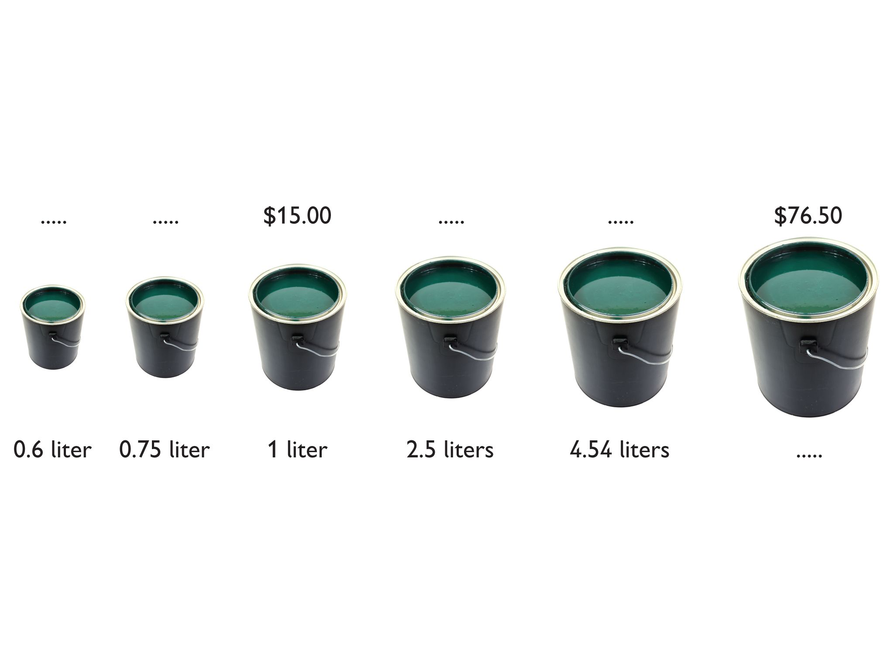Subject:
Ratios and Proportions
Material Type:
Lesson Plan
Level:
Middle School
7
Provider:
Pearson
Tags:
• Graphing
• Percentages
• Slope
Language:
English
Media Formats:
Text/HTML

# Analyzing Proportional Relationship Graphs## Overview

Students analyze the graph of a proportional relationship in order to find the approximate constant of proportionality, to write the related formula, and to create a table of values that lie on the graph.

# Key Concepts

• The constant of proportionality determines the steepness of the straight-line graph that represents a proportional relationship. The steeper the line is, the greater the constant of proportionality.
• On the graph of a proportional relationship, the constant of proportionality is the constant ratio of y to x, or the slope of the line.
• A proportional relationship can be represented in different ways: a ratio table, a graph of a straight line through the origin, or an equation of the form y = kx, where k is the constant of proportionality.

# Goals and Learning Objectives

• Identify the constant of proportionality from a graph that represents a proportional relationship.
• Write a formula for a graph that represents a proportional relationship.
• Make a table for a graph that represents a proportional relationship.
• Relate the constant of proportionality to the steepness of a graph that represents a proportional relationship (i.e., the steeper the line is, the greater the constant of proportionality).

# Lesson Guide

• Have students examine each representation (i.e., verbal description, unit rate, formula, table, and graph), drawing connections between them.

# Mathematics

The task allows students to synthesize what they have learned about proportional relationships represented in different forms: verbal descriptions, unit rates, tables, formulas, and graphs. The representations build on one another and should be connected as you move from one to the next. As students focus on each, ask:

• How does each unit price show up in the tables? (Answer: The unit price is shown in a single pair of values in each table—the pair (1, k)—and in the constant ratio between every pair of values.)
• How does each unit price show up in the formulas? (Answer: as the constant of proportionality)

ELL: When working with ELLs, it is important to link new concepts to students’ prior knowledge and experiences. This allows students to make more meaningful connections with the new mathematical concepts being learned. Remind students to review their Notebook if they are unsure how to make connections between each representation.

# The Price of Tomatoes

These ratio tables show the relationship between the cost and weight of tomatoes at two different stores.

• At Fruit World, the price for tomatoes is $0.60 per pound. The ratio is 0.6 : 1. • At Veggie Mart, the price for tomatoes is$1.15 per pound. The ratio is 1.15 : 1.
• The formula for the cost of tomatoes at Fruit World is c = 0.6w.
• The formula for the cost of tomatoes at Veggie Mart is c = 1.15w.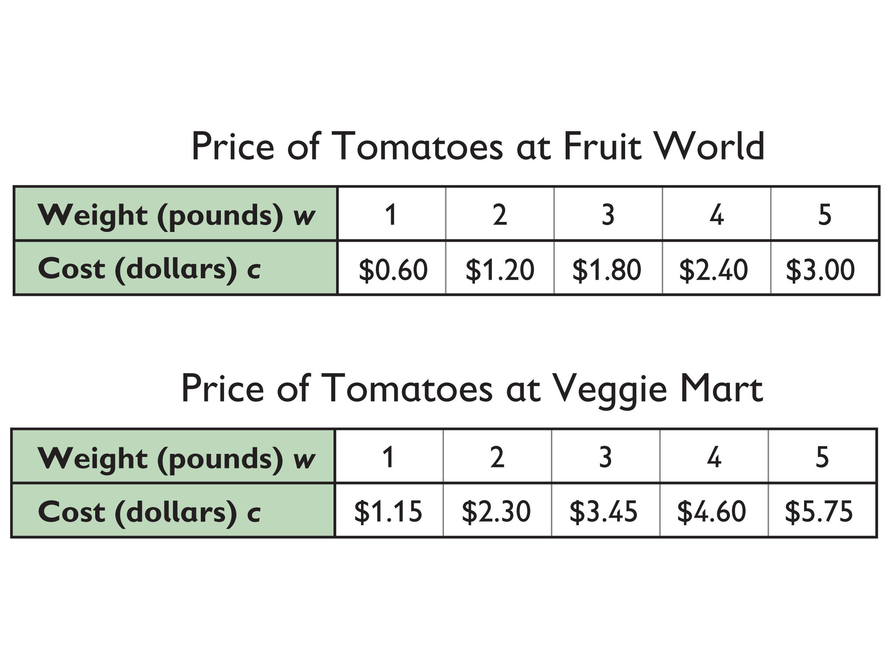# Lesson Guide

• Have partners explain how they know which graph represents each market.

# Mathematics

The representations build on one another and should be connected as you move from one to the next. As students focus on each, ask:

• How do the unit prices help you identify which graph is which? (Answer: The unit price is the constant ratio of the y-value to the x-value for the pair of values that represent a point on the line. This value can be quickly found because it is the y-value when the x-value equals 1.)

• The line that represents the cost of the tomatoes at Veggie Mart is the line containing the point (1, 1.15).
• The line that represents the cost of the tomatoes at Fruit World is the line containing the point (1, 0.6).

# Graphs

The graph shows both proportional relationships.

Fruit World: c = 0.6w

Veggie Mart: c = 1.15w

• Which line represents the cost of the tomatoes at Veggie Mart?
• Which line represents the cost of the tomatoes at Fruit World?
• Explain how you know.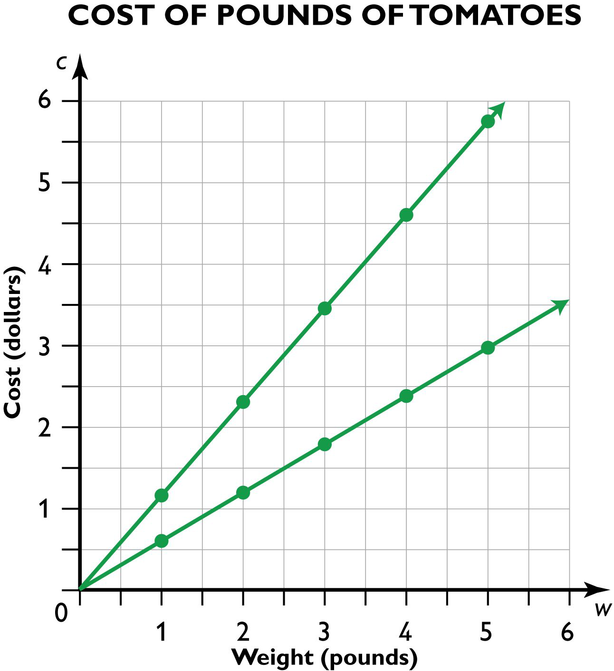# Lesson Guide

Discuss the Math Mission. Students will analyze the graph of a proportional relationship to find the approximate constant of proportionality, write the related formula, and create a table of values that lie on the graph.

SWD: Struggling students may still need explicit instruction and guided scaffolding to recognize the relationships between the constant of proportionality, table, graph, and equation. Provide small group instruction to help them make connections between the constant of proportionality and the other representations given.

## Opening

Analyze the graph, write the related formula, and create a table of values for a proportional relationship.

# Lesson Guide

Have students work in pairs for all problems and the presentation.

SWD: Prompt students with disabilities to refer to the visual representations of information in the graphs in order to make visual connections about the steepness of the lines and the value of the constant of proportionality.

# Interventions

Student does not know how to approximate the constant of proportionality from the graphs.

• Are you comparing the y-value of a particular point to the x-value of that same point to find the constant of proportionality?
• Pick any point on a graph and identify the y- and x-coordinates of that point. What is the ratio between the y- and x-coordinates? Pick another point on the same graph and do the same thing. Do you get the same ratio?

Student does not see the connection between corresponding values in a relationship and points on the graph.

• Values on the vertical axis are the y-values. Values on the horizontal axis are the x-values.
• The coordinates of each point on the graph (x, y) is a pair of corresponding values that fits the proportional relationship between the quantities represented by x and y.

Student does not see the connection between the constant of proportionality and the steepness of the graph.

• Look at the graph that is steepest. What is its constant of proportionality? Now look at the graph that is least steep. What is its constant of proportionality? Compare the two ratios.

Student thinks the endpoints of each graph determine each constant of proportionality, but does not know how to interpret them correctly.

• Pick any point on a graph and identify the x- and y-coordinates of that point. What is the ratio between the x- and y-coordinates? Now look at the endpoint shown on the same graph and do the same thing. Do you get the same ratio? Try it with another point on the same graph.

Answers may vary slightly since students are approximating from the graphs, not from specific values. Possible answers:

• Line a: $\frac{5}{2}$

Line b: $\frac{5}{4}$

Line c: $\frac{5}{6}$

Line d: $\frac{5}{11}$

Line e: $\frac{1}{3}$

• The graph of a proportional relationship with a constant of 1 would lie between lines b and c. Explanations will vary. Possible explanation: The constant of proportionality gives the steepness of the line and 1 is between $\frac{5}{4}$ and $\frac{5}{6}$, which are the constants of proportionality of lines b and c.

# Graphs of Proportional Relationships

The coordinate grid shows the graphs of five proportional relationships.

• Approximate, as closely as possible, the constant of proportionality for each line.
• The graph of a proportional relationship with a constant of 1 would lie between two of these lines. Explain why.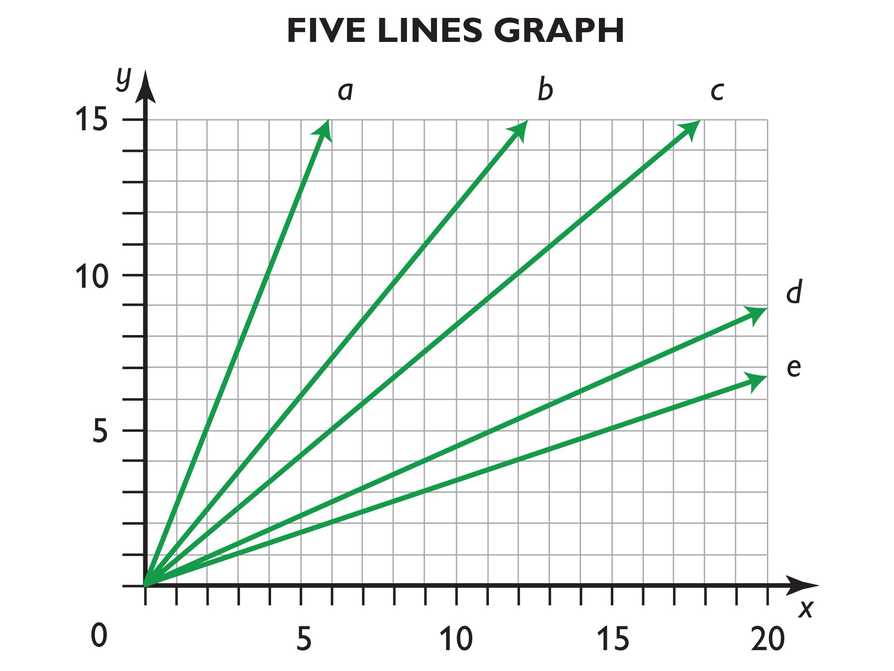## Hint:

• If you can find one point on the line, how can you determine the constant of proportionality?
• What would a line with a constant of proportionality of 1 look like? What are some examples of ordered pairs that have a ratio of 1?

# Interventions

If student does not know how to get started.

• Pick two points on the graph. How would you determine the constant of proportionality?
• What might be a good point to choose.
• What is the general form of a proportional relationships?

• Answers will vary depending on the graph students choose.

Line a: y = ($\frac{5}{2}$)x

Line b: y = ($\frac{5}{4}$)x

Line c: y = ($\frac{5}{6}$)x

Line d: y = ($\frac{5}{11}$)x

Line e: y = ($\frac{1}{3}$)x

# Write a Formula

Choose one of the lines and write the formula of the line. Use x and y to name the variables.## Hint:

Once you know the constant of proportionality, how can you use it to write the formula?

# Interventions

If student does not know how to get started.

• How could you determine one point on the graph?
• How could you determine a second point?
• What relationship can you use to generate as many points as you want?

• Answers will vary depending on the line students choose. Check that students’ tables include only positive values for x and y and include five corresponding pairs of values with the correct constant of proportionality.

# Make a Table

Choose a different line. Make a table that lists five pairs of values that define points on the graph.# Preparing for Ways of Thinking

Look and listen for students who:

• Have a range of ways of talking about the steepness of the graphs and who express uncertainty about the significance of the steepness of a graph.
• Discuss what a steeper graph means in terms of the relationship between x and y.
• Discuss what a steeper graph means in terms of the constant of proportionality.
• Observe that all of the graphs intersect at the point (0, 0).

# Challenge Problem

• Graphs will vary. Check that students’ graphs have a slope greater than 1.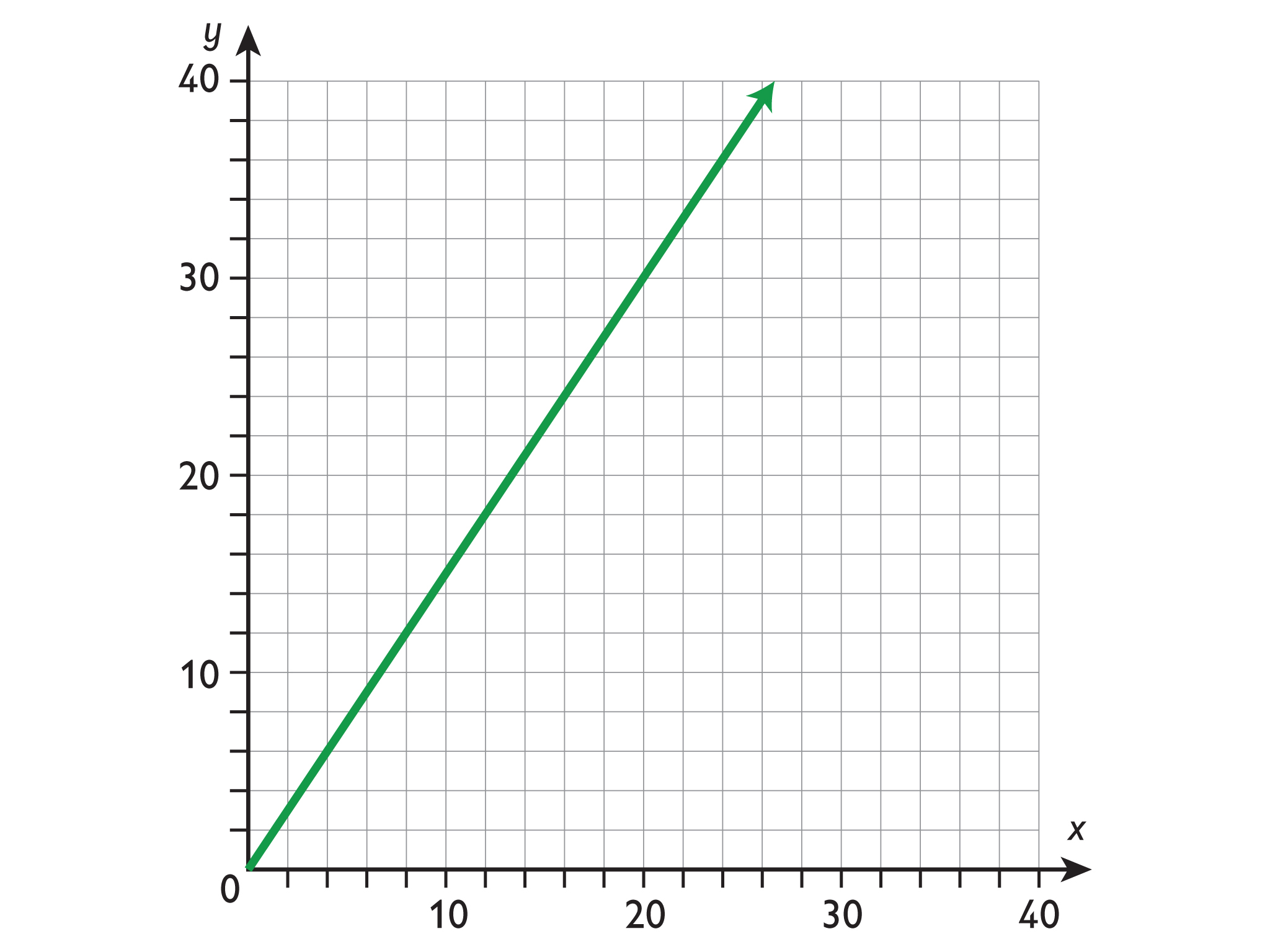# Prepare a Presentation

Prepare a presentation about what you learned about graphs and the constant of proportionality. Be sure to talk about how the constant of proportionality relates to steepness.

# Challenge Problem

Make a graph with a constant of proportionality that is greater than 1.

# Mathematics

Discuss the different formulas and tables students wrote for the different graphs.

Examine at least one case for which students have created distinct tables (with at least one distinct pair of values) for the same graph. Have students confirm that distinct tables for the same graph show the same constant of proportionality. Then make explicit the fact that many more pairs of values are possible than can be included in a table by asking:

• If you combined all of the tables created in this class for this graph, would you have all of the values on the graph? (Answer: no)
• What if you added 1,000 more pairs of values to the table? Would you have most of the values on the graph then? (Answer: no)
• Would the corresponding values all have the same constant of proportionality? (Answer: yes)

ELL: When giving directions for this discussion, be sure that students understand that they can complement their oral explanation with graphs, pictures, and drawings. Provide sentence frames that you think will be helpful for ELLs.

# Mathematical Practices

Mathematical Practice 3: Construct viable arguments and critique the reasoning of others.

• Have the class sort out any discrepancies among their constants of proportionality for each graph by giving students a chance to revise their approximations in light of others’ work. Use any discrepancies that aren’t resolved quickly as an opportunity to support students in critiquing the reasoning presented and constructing arguments about the correct constant of proportionality. Ask students to refer both to their table and to the graph as they make their cases to one another.

# Ways of Thinking: Make Connections

Take notes about your classmates’ explanations for how they found the constant of proportionality, formula, table, and to explain how the constant of proportionality relates to the steepness of a line.

## Hint:

As classmates present, ask questions such as:

• How did you find the constant of proportionality based on that graph?
• How did you figure out how to write the formula for that graph?
• What is the ratio of any two points on a line with a constant of proportionality of 1?
• How does the constant of proportionality relate to the steepness of the graph

# Mathematics

• Have pairs quietly read and then discuss the information shown.
• As student pairs work together, make a note to clarify any misunderstandings in the class discussion.
• After a few minutes, discuss the summary as a class. Ask a volunteer to explain how the constant of proportionality represents the slope of the graph that represents a proportional relationship.

Then discuss the following information with the class:

• How to find the constant of proportionality from a graph
• How to write a formula for the graph of a proportional relationship
• How to make a table to show the values that appear on a graph

SWD: When students discuss their thoughts, it provides them with opportunities to practice the thinking process involved in solving a set of problems. Listen to the students’ thought processes, correct their misconceptions, and fill in incomplete processes.

# Summary of the Math: The Slope

• In general, if quantity p is proportional to quantity q according to the formula p = kq, then the slope of the graph that represents the relationship between p and q will be equal to k, the constant of proportionality.

## Hint:

Can you:

• Explain how to find the constant of proportionality of a graph?
• Explain how to write the formula for the graph of a proportional relationship?
• Explain how to identify points on a graph, and create a table of values for a graph?
• Explain the relationship between the constant of proportionality and the steepness of the graph?

# Lesson Guide

This task allows you to assess students’ work and determine what difficulties they are having. The results of the Self Check will help you determine which students should work on the Gallery and which students would benefit from review before the assessment. Have students work on the Self Check individually.

# Assessment

Have students submit their work to you. Make notes on what their work reveals about their current levels of understanding and their different problem-solving approaches.

Do not score students’ work. Share with each student the most appropriate Interventions to guide their thought process. Also note students with a particular issue so that you can work with them in the Putting It Together lesson that follows.

SWD: Provide clear feedback to students as they attempt to solve problems or articulate concepts. This type of feedback guides students explicitly as they develop their thinking about mathematics, and gives them ideas about their next steps.

# Interventions

Student only partially records his or her computation strategies.

• Explain in more detail how you figured out your solution.

Student uses different strategies to find the missing values.

• Can you think of a solution strategy that would work for all of the missing values?

Student interprets the problem structure as additive rather than multiplicative.

• What are the two quantities that vary in relation to one another in this situation?
• Is there a constant of proportionality between the two quantities in this situation?

• Make an estimate of the answer based on how big or small you expect it to be. Does your answer fit your estimate?

Student correctly uses a unit rate to find the answers.

• Can you write a formula using the unit rate that works for finding all the missing values?
• What would you expect the graph of the relationship in this problem to look like? Identify three features you expect the graph to have.
• Sketch a graph that shows the relationship.

Student uses cross-multiplication incorrectly to find the answers.

• Identify which values in this computation are prices and which are amounts of paint: [insert a student’s calculation using cross-multiplication].
• Can you describe, in words, the relationship between the two quantities of paint in the calculation: [insert a student’s calculation using cross-multiplication]?
• Can you describe, in words, the relationship between the price and the amount of paint in this ratio: [insert one ratio from student’s work]?

Student correctly uses cross-multiplication to find the answers.

• Can you explain why this method works?
• Can you find a simpler way of calculating the missing values that works the same way in every case?

Student finds the answers correctly and efficiently.

• Find at least two different methods for finding the missing values.

1. Missing prices: $9.00,$11.25, $37.50,$68.10
Missing amount of paint: 5.1 liters

# Check the Cost of Paint

The prices are proportional to the amount of paint in the cans.

Calculate the missing prices of the paint cans and the amount of paint in the last can.